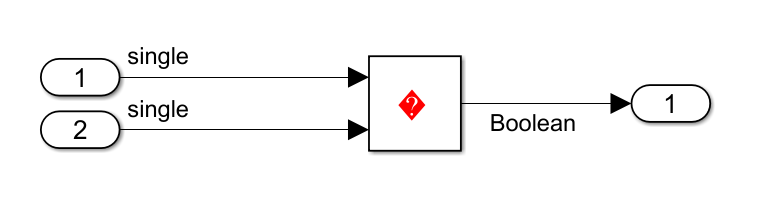# Casting modes

Method of casting data types for variables

Model Configuration Pane: Code Generation / Code Style

## Description

Specify how the code generator casts data types for variables.

## Settings

`Nominal` (default) | `Standards Compliant` | `Explicit`

Default: `Nominal`

Nominal

Generate code that uses default C compiler data type casting. This setting removes unnecessary typecasts which might lead to warnings on compilers that expect explicit casts.

Standards Compliant

Generate code where data type casting complies with MISRA™ standards.

Setting Casting modes to `Standards Compliant` can replace bitwise XOR operations with relational operations in the generated code to satisfy the MISRA C:12 Rule 10.1 when the operands are signed types.

Explicit

Generate code that casts data type values explicitly.

## Examples

expand all

Compare the data type casting in the generated code when you set Casting modes to different values.

Here is generated code that generated using the default Casting modes value `Nominal`:

```void rtwdemo_rtwecintro_step(void) { boolean_T rtb_equal_to_count; rtDWork.X++; rtb_equal_to_count = (rtDWork.X != 16); if (rtb_equal_to_count && (rtPrevZCSigState.Amplifier_Trig_ZCE != POS_ZCSIG)) { rtY.Output = rtU.Input << 1; }```

Here is the same code generated with Casting modes set to `Standards Compliant`:

```void rtwdemo_rtwecintro_step(void) { boolean_T rtb_equal_to_count; rtDWork.X = (uint8_T)((uint32_T)rtDWork.X + 1U); rtb_equal_to_count = ((int32_T)rtDWork.X != 16); if (rtb_equal_to_count && ((uint32_T)rtPrevZCSigState.Amplifier_Trig_ZCE != POS_ZCSIG)) { rtY.Output = rtU.Input << 1U; } ```

Note

The expression `rtY.Output = rtU.Input << 1U` is not compliant with MISRA C:12 Rule 10.1 because the model configuration parameter Replace multiplications by powers of two with signed bitwise shift is selected. For more information, see Replace multiplications by powers of two with signed bitwise shifts.

Depending on the setting, the configuration parameter Casting modes can replace bitwise XOR operations with relational operations in the generated code to satisfy the MISRA C:12 Rule 10.1 when the operands are signed types. For example, generate code from the following model with the Casting modes set to `Nominal` and `Standard compliant` respectively.```// Model step function (casting mode set to Nominal) void step(void) {rtY.Out3 = (boolean_T)((int32_T)(rtU.In1 != 0.0F) ^ (int32_T)(rtU.Inport1 != 0.0F)); } // Model step function (Casting modes set to Standard Compliant) void step(void) { rtY.Out3 = ((rtU.In1 != 0.0F) != (rtU.Inport1 != 0.0F)); }```
Here, the parameters `rtU.In1` and `rtU.Inport1` are single signed types. Performing a bitwise XOR(^) operation on these operands violates the MISRA C:12 Rule 10.1. To prevent this violation, the code generator replaces the bitwise XOR(^) operation with an inequality(!=) in the generated code when Casting modes is set to `Standard compliant`.

Here is the same code generated with Casting modes set to `Explicit`:

```void rtwdemo_rtwecintro_step(void) { boolean_T rtb_equal_to_count; rtDWork.X = (uint8_T)((uint32_T)(int32_T)rtDWork.X + 1U); rtb_equal_to_count = (boolean_T)((int32_T)rtDWork.X != 16); if (((int32_T)rtb_equal_to_count) && ((int32_T) rtPrevZCSigState.Amplifier_Trig_ZCE != (int32_T)POS_ZCSIG)) { rtY.Output = rtU.Input << 1; } ```

## Recommended Settings

ApplicationSetting
DebuggingNo impact
TraceabilityNo impact
EfficiencyNo impact
Safety precautionNo impact

## Programmatic Use

 Parameter: `CastingMode` Type: character vector Value: `'Nominal'` | `'Standards'` | `'Explicit'` Default: `'Nominal'`

## Version History

Introduced in R2014b